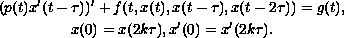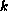Electron. J. Diff. Eqns., Vol. 2005(2005), No. 26, pp. 1-5.

### Existence of periodic solutions for second-order neutral differential equations Yongjin Li

Abstract:
By means of variational structure and critical point theory, we study the existence of periodic solutions for a second-order neutral differential equationwhereis a given positive integer andis a positive number.

Submitted January 6, 2005. Published March 6, 2005.
Math Subject Classifications: 34K13, 34K40, 65K10.
Key Words: Neutral differential equations; periodic solution; variational method; critical point.

Show me the PDF file (155K), TEX file, and other files for this article.Yongjin Li Department of Mathematics Sun Yat-sen University Guangzhou, 510275, China email: stslyj@zsu.edu.cn